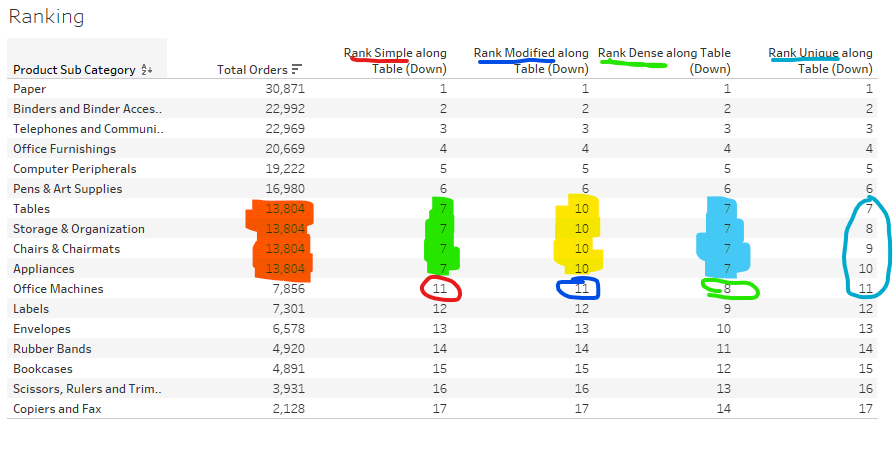# Using Rank for Comparison

Rank is a useful concept in analytics. In my experience, I have used ranking for comparing different metrics, change in performance over time, and my favorite, calculating my version of CGPA (Cumulative Grid-Point Average). In this article, we explore how to calculate rank in Excel, Power BI, and Tableau.

Mathematically speaking, rank is the position of the number in a data set, when arranged in an ascending or descending order.

# Calculating Rank in Excel

There are two functions available for calculating rank: RANK.EQ & RANK.AVG

# RANK.EQ

RANK.EQ accepts three arguments:

• Number: The number which requires ranking
• Ref: The range to compare for the ranking
• Order: 0 means ranking in descending order, 1 implies ranking in ascending order

Following table represents the output:

# RANK.AVG

The only difference is the output in the case of duplicate values, RANK.EQ assigns the higher available position to each set of duplicates and then assigns the next available position to the following value.

In the following example, RANK.EQ has assigned position 7 to Storage & Organization and Appliances, whereas rank 9 to Chair & Chairmats. It is because the 8th position/rank is not available.

RANK.AVG calculates the average of the positions and assigns it to all the duplicate sets. In the above example, it calculates the average of 7 and 8 (the result is 7.5) and assign it to the duplicate sets.

In the following example, it calculates the average of the position 7,8,9, & 10 (result is 8.5) and assigns it to the matching values:

The following table demonstrates the output of RANK.AVG, for a different number of duplicate sets available in position 7:

Pivot also comes with a feature to get the Rank. Refer to the following article for more details:

Do more with Pivot Tables: Value Field Settings

# Rank in Tableau

Calculating rank in Tableau is quite simple. We get the following formulas for the rank calculation: RANK, RANK_DENSE, RANK_MODIFIED, RANK_UNIQUE

The syntax for all the above functions is the same:

1 > Define the name of the calculated field

2 > Expression: Value for the sorting

3 > ‘Order’ > Sorting Order. This argument is optional. If not defined, Tableau applies ‘desc’ as the default order.

Let us use the first rank function: RANK

It is equivalent to RANK.EQ function in Excel

Output:

# Multi-Level Ranking

When we add Product Category in the visual, then we can have two levels of Ranking: Overall & Product Sub-category level

Drag the Rank Orders field again under Measure Values.

Click on the option arrow > Compute Using > Product Sub-Category

Apart from RANK, we have the following rank functions in Tableau:

· RANK_MODIFIED: Equivalent to the RANK function except for the way it handles the ranking of identical values. The RANK function assigns the first position to all identical values. Whereas, the RANK_MODIFIED assigns the last position.

In the example below, the identical values are from position 7 to 10. RANK assigns 7 as rank value, whereas RANK_MODIFIED assigns 10.

· RANK_DENSE: Identical values are assigned an identical rank, but it does not insert gaps into the number sequence.

· RANK_Unique: Identical values are assigned different ranksDifferent output of Rank functions in Tableau

# Calculating Rank in Power BI or Power Pivot using DAX (RANKX)

Although, DAX has RANK.EQ which is equivalent to RANK.EQ formula in Excel, we do not use it due to the limited capabilities. Instead, we use RANKX.

`Rank (Prod Sub Cat) =  IF(  //HASONEVALUE helps in getting rid of rank value under Total    HASONEVALUE(dtOrders[Product Sub-Category]),    RANKX(  //Defining the table/column for the scope of the formula    ALLSELECTED(dtOrders[Product Sub-Category]),  //the value to be sorted on        [Total Orders]      ) )`

RANKX formula has the following arguments

## <Table>

The first argument <Table> can be a physical table, or it can be an output of a DAX query that returns a table. RANKX is an iterator, and <Table> argument defines the range in which it runs the expression. About the Excel formula, it is the <ref> argument.

## <Expression>

We can read this parameter as “sort by” expression. About the Excel formula, it is the <number> argument.

## <Value>

It is an optional argument and a tricky one. To keep it simple, if we are looking for simple ranking as an output, we do not need this. We can leave this as blank.

## <Order>

It is also an optional argument. If not defined, DAX sort in descending order. We can select ASC for ascending order sorting and DESC for descending order sorting.

<Ties>

Another optional argument. The name is kind of misleading as it does not do anything for the duplicate sets. Instead, it decides whether we want to assign the next available position (SKIP) or assign next numerical ranking (DENSE)

Difference between Dense & Skip option in RANKX

The <Table> argument decides the scope of the rank function. For example, the previous DAX measure only considers the Product Sub-categories selected in the visual. The ranking updates according to the selection of Product Sub-categories:

If we include the parent hierarchy in the scope, then we can retain the original ranking, even when the selection changes:

`Rank (Prod Sub Cat) OverAll =  IF(  RANKX(  //Removing the filter context applied on Product Category & Sub-category  ALL(dtOrders[Product Category],dtOrders[Product Sub-Category]),  [Total Orders]   ) )`

Using Matrix visual to represent two different rankings:

One concept, three tools!

--

--## @imVivRan

Founder — VIVRAN.IN || BI Consultant || Trainer || Power BI Super User || Power Apps Developer || Excel Expert || www.vivran.in